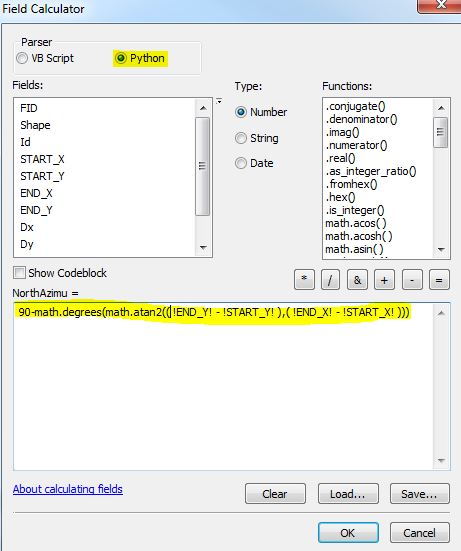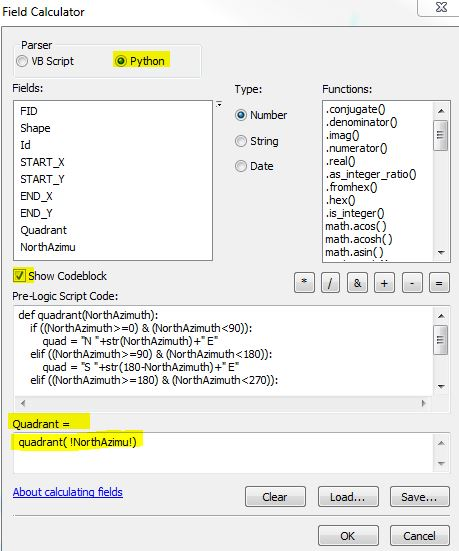English

# How To: Batch calculate line direction using the Field Calculator

## Summary

The direction of multiple line features can be calculated from their start and end point coordinates by using the Field Calculator.

## Procedure

The instructions provided describe how to calculate the line direction of multiple features using a batch process.

1. In the attribute table of the line features, add four new fields: START_X, START_Y, END_X, and END_Y. Set the field type for these fields to Double.
2. Use the Calculate Geometry function to calculate the X and Y coordinates of the line's start and end position into the four new fields.
3. Add another new field to the attribute table and name this field NorthAzimu. Set the field type to Double.
4. Calculate the NorthAzimu field with the following Python expression. Refer to the code and image below.
`90-math.degrees(math.atan2(( !END_Y! - !START_Y! ),( !END_X! - !START_X! )))`5. To convert from North Azimuth to Quadrant Bearing, add another new field: Quadrant; set the data type to String.
6. Calculate the Quadrant field by using the Python code block, as seen below. Refer to the image and ensure each item is highlighted appropriately in the Field Calculator window before running the code.
```def quadrant(NorthAzimuth):
if ((NorthAzimuth>=0) & (NorthAzimuth<90)):